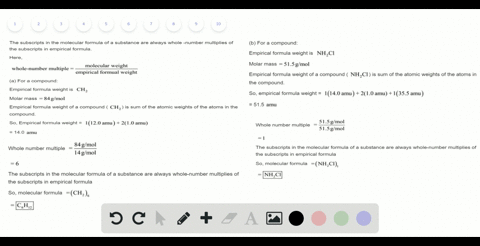Enroll in one of our FREE online STEM summer camps. Space is limited so join now!View Summer Courses### What is the molecular formula of each of the foll…

01:29Auburn University Main Campus

Need more help? Fill out this quick form to get professional live tutoring.

Get live tutoring
Problem 50

The compound $\mathrm{XCl}_{4}$ contains 75.0$\% \mathrm{Cl}$ by mass. What is the element $\mathrm{X}$ ?

Ti

## Discussion

You must be signed in to discuss.

## Video Transcript

So you're given a compound which has a formal off exhale for and here the seal of the chlorine has a percentage off seventy five percent by month. Where to find our day element X So we will start by zooming this seventy five percent as seventy five gram massive florine And from there we will find out the number ofthe most ofthe chlorine. And then we will ah use their number off malls To find out the number of malls for X and from the number of malls off eggs, we'LL be able to find out which Element X is. So let's start with as zooming seventy five percent as seventy five grams Florian that means mall's off chlorine in this compound is seventy five grams over thirty five point four five Grandpa, our mole and it will give us a value off two point one two more. So this is the number of most ofthe chlorine. Now, if we consider the mass ofthe X, um in the calm bone as twenty five grams since the total mass off the come believe one hundred gram and since Clarence Mother's seventy five grand that made the rest will be there mass off Ex says there is no other element in this compound that Mintz the mass of eggs in this compound will be twenty five grams. That means mall's off sale will be up, not sail if x Well, maybe twenty five gram over the molar mass effects which we do not know. So we will assume it as why Grandpa, our mole and well as you The number of malls as zimmel. Now what we'LL do is so sins the number ofthe chlorine atoms. You know what a molecule is for? And we know that, um, to find out the simplest ratio. What we do is we divide each number off malls. Bye. Smallest number of Malta get the simplest ratio. And since here the ratio of X is one. That means each number ofthe mall was divided by the number of malls. Off eggs to find are the simplest precio. And this and the issue of chlorine here is for which is so the ratio off X and seal here is one is two four and that means ah, Florence number of malls, which is two point one toe over the number of more off, see the number one ofthe X, which is seem all well, give us a fellow for no. And from here we can find out the Vela off. See that, Mr Bellows? He will be two point one toe more over four and give us a value off zero point five three more. So this is the number of malls off X And now just replacing C by that velo, we can find out the value ofthe way. Men's Why over? I don't know what twenty five grams over why, Grandpa, our mole is on the value of Z, which is the urban five three mall. And from here we can find a bell off. Why? Which is twenty five gram over zero point five three mall and you give us a bell off forty seven point one seven. Grandpa Armel. So this is the number. This's the molar mass off eggs. And if we compared the molar mass with that with the values are the calculated fellows will see that this is close. Toe the monuments off titanium, which has a mother myself off forty seven point eight seven gram bar. More so from Erica's say that the Element X is titanium